# Excel MID function to extract text from the middle of a string

MID is one of the Text functions that Microsoft Excel provides for manipulating text strings. At the most basic level, it is used to extract a substring from the middle of the text string. In this tutorial, we will discuss the syntax and specificities of the Excel MID function, and then you will learn a few creative uses to accomplish challenging tasks.

## Excel MID function - syntax and basic uses

Generally speaking, the MID function in Excel is designed to pull a substring from the middle of the original text string. Technically speaking, the MID function returns the specified number of characters starting at the position you specify.

The Excel MID function has the following arguments:

MID(text, start_num, num_chars)

Where:

• Text is the original text string.
• Start_num is the position of the first character that you want to extract.
• Num_chars is the number of characters to extract.

All 3 arguments are required.

For example, to pull 7 characters from the text string in A2, starting with the 8th character, use this formula:

`=MID(A2,8, 7)`

The result might look something similar to this: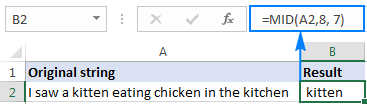### 5 things you should know about Excel MID function

As you have just seen, there's no rocket science in using the MID function in Excel. And remembering the following simple facts will keep you safe from most common errors.

1. The MID function always returns a text string, even if the extracted substring contains only digits. This may be critical if you wish to use the result of your Mid formula within other calculations. To convert an output into a number, use MID in combination with the VALUE function as shown in this example.
2. If start_num is greater than the overall length of the original text, an Excel Mid formula returns an empty string ("").
1. If start_num is less than 1, a Mid formula returns the #VALUE! error.
2. If num_chars is less than 0 (negative number), a Mid formula returns the #VALUE! error. If num_chars is equal to 0, it outputs an empty string (blank cell).
3. If the sum of start_num and num_chars exceeds the total length of the original string, the Excel MID function returns a substring starting from start_num and up to the last character.

## Excel MID function - formula examples

When dealing with real-life tasks in Excel, you will most often need to use MID in combination with other functions as demonstrated in the following examples.

### How to extract first and last name

If you've had a chance to read our recent tutorials, you already know how to pull the first name using the LEFT function and get the last name with the RIGHT function. But as is often the case in Excel, the same thing can be done in a variety of ways.

#### MID formula to get the first name

Assuming the full name is in cell A2, first and last names separated with a space character, you can pull the first name using this formula:

`=MID(A2,1,SEARCH(" ",A2)-1)`

The SEARCH function is used to scan the original string for the space character (" ") and return its position, from which you subtract 1 to avoid trailing spaces. And then, you use the MID function to return a substring beginning with the fist character and up to the character preceding the space, thus fetching the first name.

#### MID formula to get the last name

To extract the last name from A2, use this formula:

`=TRIM(MID(A2,SEARCH(" ",A2),LEN(A2)))`

Again, you use the SEARCH function to determine the starting position (a space). There is no need for us to calculate the end position exactly (as you remember, if start_num and num_chars combined is bigger than the total string length, all remaining characters are returned). So, in the num_chars argument, you simply supply the total length of the original string returned by the LEN function. Instead of LEN, you can put a number that represents the longest surname you expect to find, for example 100. Finally, the TRIM function removes extra spaces, and you get the following result: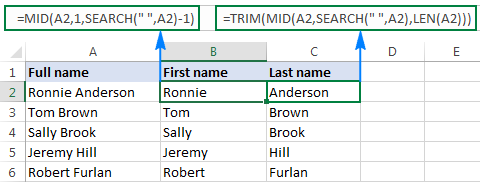Tip. To extract the first and last word from a string with a simpler formula, you can use the custom ExtractWord function.

### How to get substring between 2 delimiters

Taking the previous example further, if besides first and last names cell A2 also contains a middle name, how do you extract it?

Technically, the task boils down to working out the positions of two spaces in the original string, and you can have it done in this way:

• Like in the previous example, use the SEARCH function to determine the position of the first space (" "), to which you add 1 because you want to start with the character that follows the space. Thus, you get the start_num argument of your Mid formula: SEARCH(" ",A2)+1
• Next, get the position of the 2nd space character by using nested Search functions that instruct Excel to start searching from the 2nd occurrence of the space character: SEARCH(" ",A2,SEARCH(" ",A2)+1)

To find out the number of characters to return, subtract the position of the 1st space from the position of the 2nd space, and then subtract 1 from the result since you don't want any extra spaces in the resulting substring. Thus, you have the num_chars argument: SEARCH (" ", A2, SEARCH (" ",A2)+1) - SEARCH (" ",A2)

With all the arguments put together, here comes the Excel Mid formula to extract a substring between 2 space characters:

=MID(A2, SEARCH(" ",A2)+1, SEARCH (" ", A2, SEARCH (" ",A2)+1) - SEARCH (" ",A2)-1)

The following screenshot shows the result: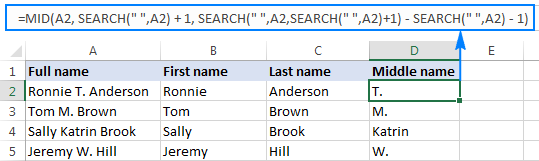In a similar manner, you can extract a substring between any other delimiters:

MID(string, SEARCH(delimiter, string)+1, SEARCH (delimiter, string, SEARCH (delimiter, string)+1) - SEARCH (delimiter, string)-1)

For example, to pull a substring that is separated by a comma and a space, use this formula:

=MID(A2,SEARCH(", ",A2)+1,SEARCH(", ",A2,SEARCH(", ",A2)+1)-SEARCH(", ",A2)-1)

In the following screenshot, this formula is used to extract the state, and it does the job perfectly: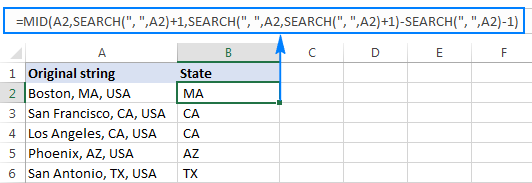### How to extract Nth word from a text string

This example demonstrates an inventive use of a complex Mid formula in Excel, which includes 5 different functions:

• LEN - to get the total string length.
• REPT - repeat a specific character a given number of times.
• SUBSTITUTE - replace one character with another.
• MID - extract a substring.
• TRIM - remove extra spaces.

The generic formula is as follows:

TRIM(MID(SUBSTITUTE(string," ",REPT(" ",LEN(string))), (N-1)*LEN(string)+1, LEN(string)))

Where:

• String is the original text string from which you want to extract the desired word.
• N is the number of word to be extracted.

For instance, to pull the 2nd word from the string in A2, use this formula:

`=TRIM(MID(SUBSTITUTE(A2," ",REPT(" ",LEN(A2))), (2-1)*LEN(A2)+1, LEN(A2)))`

Or, you can input the number of the word to extract (N) in some cell and reference that cell in your formula, like shown in the screenshot below: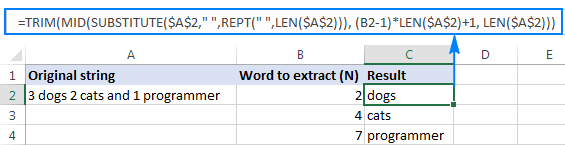#### How this formula works

In essence, the formula wraps each word in the original string with many spaces, finds the desired "spaces-word-spaces" block, extracts it, and then removes extra spaces. To be more specific, the formula works with the following logic:

• The SUBSTITUTE and REPT functions replace each space in the string with multiple spaces. The number of additional spaces is equal to the total length of the original string returned by LEN: SUBSTITUTE(A2," ",REPT(" ",LEN(A2)))

You can think of an intermediate result as of "asteroids" of words drifting in space, like this: spaces-word1-spaces-word2-spaces-word3-… This "spacious" string is supplied to the text argument of our Mid formula.

• Next, you work out the starting position of the substring of interest (start_num argument) using the following equation: (N-1)*LEN(A1)+1. This calculation returns either the position of the first character of the desired word or, more often, the position of some space character in the preceding space separation.
• The number of characters to extract (num_chars argument) is the easiest part - you simply take the overall length of the original string: LEN(A2). At this point, you are left with spaces-desired word-spaces substring.
• Finally, the TRIM function gets rid of leading and trailing spaces.

The above formula works fine in most situations. However, if there happen to be 2 or more consecutive spaces between words, it yields wrong results. To fix this, nest another TRIM function inside SUBSTITUTE to remove excess in-between spaces except for a single space character between words, like this:

`=TRIM(MID(SUBSTITUTE(TRIM(A2)," ",REPT(" ",LEN(A2))), (B2-1)*LEN(A2)+1, LEN(A2)))`

The following screenshot demonstrates the improved formula in action: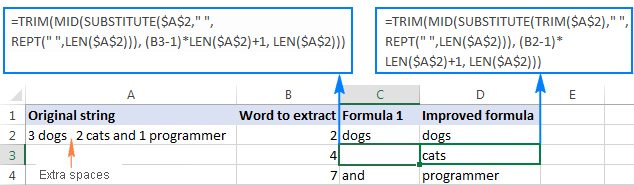If your source strings contain multiple spaces between words as well as very big and very small words, additionally embed a TRIM function into each LEN, just to keep you on the safe side:

`=TRIM(MID(SUBSTITUTE(TRIM(A2)," ",REPT(" ",LEN(TRIM(A2)))), (B2-1)*LEN(TRIM(A2))+1, LEN(TRIM(A2))))`

I agree that this formula looks a bit cumbersome, but it impeccably handles all kinds of strings.

Tip. See how to extract any Nth word from text using a more compact and straightforward formula.

### How to extract a word containing a specific character(s)

This example shows another non-trivial Excel Mid formula that pulls a word containing a specific character(s) from anywhere in the original text string:

TRIM(MID(SUBSTITUTE(string," ",REPT(" ",99)),MAX(1,FIND(char,SUBSTITUTE(string," ",REPT(" ",99)))-50),99))

Assuming the original text is in cell A2, and you are looking to get a substring containing the "\$" character (the price), the formula takes the following shape:

`=TRIM(MID(SUBSTITUTE(A2," ",REPT(" ",99)),MAX(1,FIND("\$",SUBSTITUTE(A2," ",REPT(" ",99)))-50),99))`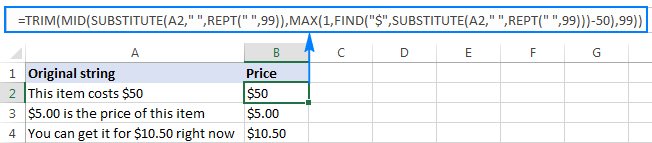In a similar fashion, you can extract email addresses (based on the "@" char), web-site names (based on "www"), and so on.

#### How this formula works

Like in the previous example, the SUBSTITUTE and REPT functions turn every single space in the original text string into multiple spaces, more precisely, 99 spaces.

The FIND function locates the position of the desired character (\$ in this example), from which you subtract 50. This takes you 50 characters back and puts somewhere in the middle of the 99-spaces block that precedes the substring containing the specified character.

The MAX function is used to handle the situation when the desired substring appears in the beginning of the original text string. In this case, the result of FIND()-50 will be a negative number, and MAX(1, FIND()-50) replaces it with 1.

From that starting point, the MID function collects the next 99 characters and returns the substring of interest surrounded by lots of spaces, like this: spaces-substring-spaces. As usual, the TRIM function helps you eliminate extra spaces.

Tip. If the substring to be extracted is very big, replace 99 and 50 with bigger numbers, say 1000 and 500.

### How to force an Excel Mid formula to return a number

Like other Text functions, Excel MID always returns a text string, even if it contains only digits and looks much like a number. To turn the output into a number, simply "warp" your Mid formula into the VALUE function that converts a text value representing a number to a number.

For example, to extract a 3-char substring beginning with the 7th character and convert it to a number, use this formula:

`=VALUE(MID(A2,7,3))`

The screenshot below demonstrates the result. Please notice the right-aligned numbers pulled into column B, as opposed to the original left-aligning text strings in column A: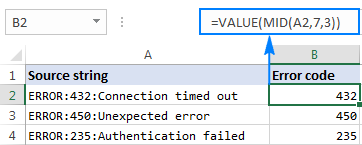The same approach works for more complex formulas as well. In the above example, assuming the error codes are of a variable length, you can extract them using the Mid formula that gets a substring between 2 delimiters, nested within the VALUE function:

`=VALUE(MID(A2,SEARCH(":",A2)+1,SEARCH(":",A2,SEARCH(":",A2)+1)-SEARCH(":",A2)-1))`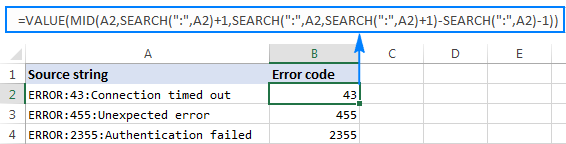This is how you use the MID function in Excel. To better understand the formulas discussed in this tutorial, you are welcome to download a sample workbook below. I thank you for reading and hope to see you on our blog next week!

Excel MID function - formula examples (.xlsx file)

## More examples of using the MID function in Excel:

1. Hi i have one query, I am unable to find the total months for the age 17:00 ,
Can any one help me

2. Hello, this thread has been very helpful. I have a column where wages are listed among other text. In some cells it is a static amount, in others it is a range. Example: "Regular Full-time, 40 hours/week \$24.35 - \$27.55 Hourly"
I have used a LEN formula to figure out how many \$ symbols there are in Column F and am using an if formula to return the wage if only one \$ is present, but I am having trouble returning only the range. I do not want "Hourly". The ideal result would be "\$24.35 - \$27.55".
Here is the formula I am using, but it only returns "\$24.35".
=IF(F14=1,TEXTBEFORE(TEXTAFTER(E14,"\$")," "),TRIM(MID(SUBSTITUTE(E14," ",REPT(" ",99)),MAX(1,FIND("\$",SUBSTITUTE(E14," ",REPT(" ",99)))-50),99)))

• Use substring functions to extract the desired result from the text. Try this formula:

=MID(E14, SEARCH("\$",E14), (SEARCH(" ",E14,SEARCH("#",SUBSTITUTE(E14,"\$","#",2))))-SEARCH("\$",E14))

Hope this is what you need.

3. 3459MohamedMO
5648SalahSA
5696YoussefYO
4167MahmoudMA
1236EmanEM

i have a whole column like that and I want to extract the middle name out of the first 4 numbers and the last 2 letters
how to get that? and how to use MID() in this case?
the needed output:
Mohamed
Salah
Youssef
MAhmoud
Eman

• Hi! Find the number of characters to be extracted using the LEN function. Please check the formula below, it should work for you:

=MID(A1,5,LEN(A1)-6)

4. Hi,

I need to find the exact position of the cell A1= DBR from:
cell A2 = DW=4000 DH=2100 TT=1 TTT=68 M=2 MDBR=0 EH=1 EC=1 DTR=0 DBR=50

When I use =FIND(A1,A2) it will return me position of DBR from MDBR which is the first parameter containg DBR in the name, but after that we have DBR in the end which I am interested in.

Which formula will be the best to extract exact name from A1.

Thanks

• Hi! Try adding a space to the search string:

=FIND(" "&A1,A2)

5. Good Evening, appreciate if anyone on the thread is able to assist.

The following is an example of the raw data, Whereby OM = Original; CH = Chocolate; CS = Chia Seed; and the number preceding is the total quantity of product - if no number is stated, it is simply 1 X of the stated product.
OMCHCS
2OM2CH
2CS2CS
2CH1OM

What I require is to split this data into columns (Name of Product followed by Quantity of Product).
E.g. for the 1st line (OMCHCS):
Cell A1: Original
Cell A2: 1
Cell B1: Chocolate
Cell B2: 1
Cell C1: Chia Seed
Cell C2: 1

E.g. for the 2nd line (2OM2CH)
Cell A1: Original
Cell A2: 2
Cell B1: Chocolate
Cell B2: 2
Cell C1: Chia Seed
Cell C2: 0

Would this be possible using simply formulas in excel?
Thank you.

• Hi! Your OMCHCS and 2OM2CH data are in different formats and cannot be split to cells using the same formula. For the text string OMCHCS:

=MID(D1,1,2)
=MID(D1,3,2)
=MID(D1,5,2)

To convert "OM" to "Original" you can use the IFS function or extract "Original" from the matching table using the VLOOKUP or INDEX MATCH formula. For example:

=IFS(MID(D1,1,2)="OM","Original", MID(D1,1,2)="CH","Chocolate")

6. Hi Sir,
NEFT-HSBCN23212775696-NATIONAL INSURANCE CO LTD.
How can I find out HSBCN23212775696 from this, which formula will I use?

Thanks

• Hello! I’m not sure I got you right since the description you provided is not entirely clear. If you want to determine a partial match of text strings, use these instructions: How to find substring in Excel.
If you want to extract the text, use this guide from the article above: How to get substring between 2 delimiters.

7. sir i have doubt thats sa@07gar07 into sagar@0707 into dynamic whats the formula.

• Hi! Extract separate text strings using the MID function and combine them using the & operator. Please read the above article carefully.

=MID(A1,1,2)&MID(A1,6,3)&MID(A1,3,3)&MID(A1,9,2)

8. Hello, and thank you for your dedication, I have a cell with about 20 lines of data, from there I only want to extract 4 digits after the semicolon on the 10th line and nothing after. Data can look like; I get it to work with =TEXTAFTER(D4,Area Code: #") but i cannot get it to stop after those three digits and pull everything after..

City: Miami
State: FL
Town: anything
Area Code: # 305
Province:
Country: US
Work Location: Local

• Hi! Your question does not match the formula and data you wrote below. If you want to extract the first 4 characters from the text, use the LEFT function. For example,

=LEFT(TEXTAFTER(D4,"Area Code: #"),4)

• Thank you! that worked perfectly! i guess i was rushing trying to explain.

9. Hello, I have a string like this

SEP 28 SQUARESPACE INC. HTTPSSQUARES \$46.61

And I need to remove the date on the left and the price of transaction on the right. Is that something I can do in one formula?

• Hi! Use TEXTBEFORE and TEXTAFTER functions to get the text string before and after a certain character. Find the number of the last space in the text as recommended in this article.

=TEXTAFTER(TEXTBEFORE(A1," ",LEN(A1) - LEN(SUBSTITUTE(A1," ","")))," ",2)

10. Hi,
Im looking to extract the 7 digit number starting with 3 in a cell and place it in a cell on its own. The number changes in the string. It always starts with a 3 and has 7 digits.
Below is an example:

INV-8757 16/06/20 21591 1 x Carton (51 x 31 x 50cm @ 78Kg) PO 3115158 - 1 x Carton 1 x Valve
INV-8757 16/06/20 21593 1 x Pallet (60 x 55 x 25cm @ 124KG)PO 3114189 - 1 x Pallet
INV-8757 16/06/20 21594 1 x Box (20 x 15 x 25cm @ 1KG)PO 3115188 - delivery

• To find text based on a pattern, you can use regular expressions and the custom RegExpExtract function. You can find the examples and detailed instructions here: How to extract substrings in Excel using regular expressions (Regex). The formula might look like this:

=RegExpExtract(A2, "3\d{6}", 1)

I recommend paying attention to the Regex tool. You can find, extract, compare, delete, or replace strings that match the regular expression pattern you enter. It is available as a part of our Ultimate Suite for Excel that you can install in a trial mode and check how it works for free.

11. Order No: Prices:
AB124 3.20
AB124 3.10
AB124 3.87
AB124 3.45
CD245 3.20
BQ458 3.10

In the above scenario, How to pick only AB124's 2nd and 3rd value?

• Hi! If I understand your task correctly, pay attention to the following paragraph of the article above: MID formula to get the last name.

12. I have this email script in my first colum A1:

"We are glad to hear from you again.

Please ensure that you account is being activated before the due date.

Here is your user ID abcdefg123@popit.com"

My question:
Different customers would have different user ID
How do I trim the login ID (abcdefg123@popit.com) to be appeared in another column : B1.

Thanks :)

• To extract the last word from a text, you can use the TEXTAFTER function. Try this formula:

=TEXTAFTER(A1," ",-1)

You can also find the examples and detailed instructions here: How to extract Nth word from a text string.

=TRIM(MID(SUBSTITUTE(A1," ",REPT(" ",LEN(A1))), (LEN(A1)-LEN(SUBSTITUTE(A1," ",""))) * LEN(A1)+1, LEN(A1)))

13. Please support getting the text "RET-EXPIRED", "Expired", and "DAMAGES" only from the below text in excel.

P-02-RET-EXPIRED
P-07-Expired-2023
P-07-DAMAGES-2023

• Hi! You can try to find these text strings in the cells and display the corresponding message. Use this guide: How to find substring in Excel. For example:

=IF(ISNUMBER(SEARCH("RET-EXPIRED",A1)),"RET-EXPIRED", IF(ISNUMBER(SEARCH("DAMAGES",A1)),"DAMAGES",""))

You can to use nested IF function.

14. Hi Sir,

FLAT/ROOM 2504, FLOOR 25, TONG BO HOUSE, FU TONG ESTATE, TONG CHONG
FLAT/RM 18 5/F TSU YONG HSE TSU PONG NORTH ESTATE
FLAT/RM 19 13/F MAI SHAK HOUSE SHAK MEN ESTATE
FLAT/ROOM 2103, FLOOR 21, LENG CUN HOUSE, LENG KING ESTATE
FLAT 05 15/F IN CHING HOUSE UN CHUNG ESTATE
FLAT/RM 3106, 31/F, MAN KING HOUSE, TSZ MING ESTATE
ROOM 2428, MIND SHIN HSE, MING SHING ESTATE
FLAT 1019, FLOOR 10, MEN KONG HOUSE, MODEL ESTATE
FLAT/ROOM 1106, 11/F YAN YUE HOUSE, TENG HANG ESTATE

I want to find the starting position of XX HOUSE by looking two " " to the left of "HOUSE" in order to extract "X X HOUSE" from the string

• Thank you for you reply,
However, I cannot find textsplit in my Excel 2016.

15. I have a very descriptive Cell (2000+ in the column). I want to extract from each cell User: John Smith and user Certifcate Serial Number in two separate columns. How do I achieve this:

The Key Distribution Center (KDC) encountered a user certificate that was valid but could not be mapped to a user in a secure way (such as via explicit mapping, key trust mapping, or a SID). The certificate also predated the user it mapped to, so it was rejected. See http s://go.microsoft.com/fwlink/?linkid=2189925 to learn more.

User: John Smith
Certificate Subject: @@@OID.0.9.2242.17200300.102.1.1=12008002283899 CN=JOHN SMITH (Affiliate), OU=Support Office, O=Microsoft, C=US
Certificate Issuer: Digicert CA
Certificate Serial Number: 6109AE1K
Certificate Thumbprint: 75024E979EA9006B1770
Certificate Issuance Time: 13113180000000
Account Creation Time: 1332200000000

• Hi! Try to use new Excel functions TEXTBEFORE and TEXTAFTER.
For example:

=TEXTBEFORE(TEXTAFTER(A1,"User:"),CHAR(10))

16. Here the below two sentence given. first sentence has word OBI= so I used formula to extract details start from ORG= till OBI=
The second sentence doesn't have word OBI= so please advise me how to use formula to take full details from ORG= if no OBI= word found.
If OBI= found, then details should come till OBI= from ORG=
if no OBI= not found then details should take all details from ORG=

Sentence 1 - TRSF BOOK TRANSFER CREDIT SND=NOREF ORG=RSB IN OBI=ATTN/RSB
Sentence 2 - TRSF BOOK TRANSFER CREDIT SND=NOREF ORG=CAIRE CONSULT IN

The formula I used for sentence 1 is =MID(D1,FIND("ORG=",D1)+0,FIND("OBI=",D1)-FIND("ORG=",D1)+4)

• Hi! If I understand your question correctly, your formula can be used in the IFERROR function. If "ORG=" is not found and an error is received, all characters until the end of the text string are extracted.

=IFERROR(MID(D1,FIND("ORG=",D1)+0,FIND("OBI=",D1)-FIND("ORG=",D1)+4), MID(D1,FIND("ORG=",D1)+0,100))

17. Sir,
As we know MID function returns a string value . without using VALUE function i got numerical output for =(MID(E5,1,1)+MID(E5,2,1)+MID(E5,3,1) this formula instead of concatenating . can you please explain how is that possible.